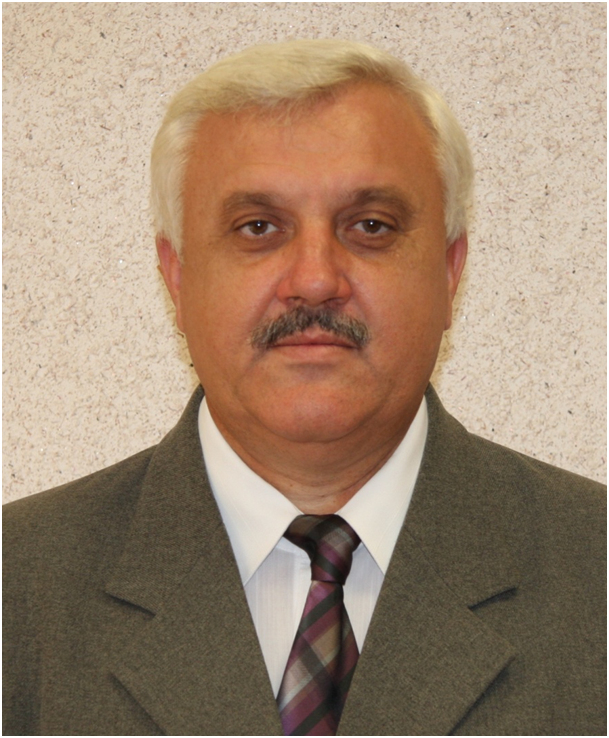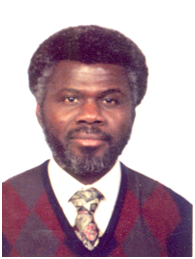# IT.I1.STATISTICS.0.ExProbability theory and mathematical statistics

This notebook aims to foster the theory of both probability and statistical inference for undergraduate students in statistics or other areas in which a good understanding of statistical concepts is essential.
False
registered False
in_cart False
is_course_full False

invitation_only False
can_enroll True

The first two chapters introduce the basic concepts and techniques in probability theory, including the calculus of probability, conditional probability, independence of events, Bayes's Theorem. The next three chapters contain the concepts of the random variables, probability distributions, transformations, expectation, moments, the most important discrete and continuous distributions and the properties of these concepts. Chapter 6 develops the notions of multivariate random variables (random vectors), independence of random variables, conditional distributions, conditional expectation and the basic concepts of random simulation. Chapter 7 contains some mixed areas: standard probability inequalities, law of large numbers and the central limit theorem.

The remainder of the notes systematically develops the concepts of statistical inference. The sample, statistics are defined in Chapter 8. This chapter contains the basic statistics and Glivenko's theorem. The point estimator, the confidence interval, the Cramer-Rao inequality, the method of moment estimator, maximum likelihood estimator are developed in Chapter 9. Two-sided alternative hypotheses, likelihood ratio and chi squared tests are developed in Chapter 10. Chapters 9 and 10 present the basic ideas of large sample confidence intervals and test procedures.

## prerequisites

The prerequisite is one year's worth of calculus. That should be enough to understand a major portion of the notes. There are sections for which some familiarity with linear algebra, multiple integration and partial differentiation will be beneficial.

## Course Staff### Dr.Fegyverneki Sándor

Qualification: applied mathematician and english-hungarian translator, KLTE, 1984. Current employment:University of Miskolc, Department of Applied Mathematics. Scientific degree: PhD, mathematics and computer science (probability theory and mathematical statistics), 2001. Teaching skills: probability theory, mathematical statistics, information theory, numerical methods, optimization, programming theory.### Dr. Karácsony Zsolt

Qualification: mathematician, University of Debrecen, 2003. Current employment:University of Miskolc, Department of Applied Mathematics. Scientific degree: PhD, mathematics and computer science (probability theory and mathematical statistics), 2011. Teaching skills: probability theory, mathematical statistics, numerical methods, optimization, programming theory.### Dr. Nutefe Kwami Agbeko

Qualification: mathematician, ELTE, 1982. Current employment:University of Miskolc, Institute of Mathematics. Scientific degree: PhD, mathematics and computer science (probability theory and mathematical statistics), 2009. Teaching skills: probability theory, mathematical statistics, applied linear algebra, numerical methods, optimization, operation research etc.

## FAQ

### Do I need to buy a textbook?

No, a free online version of Chemistry: Principles, Patterns, and Applications, First Edition by Bruce Averill and Patricia Eldredge will be available, though you can purchase a printed version (published by FlatWorld Knowledge) if you’d like.